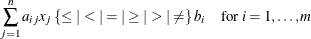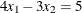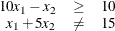### LINCON Statement

LINCON linear_constraint-1 <…,linear_constraint-n> ;

LINEAR linear_constraint-1 <…,linear_constraint-n> ;

where linear_constraint has the form

linear_expression-type linear_expression-where linear_expression has the form

<+|->linear_term-1 <…, (+|-) linear_term-n>

where linear_term has the form

(variable | number<* variable>)

The keyword type can be one of the following:

<, <=, =, >=, >, <>, LT, LE, EQ, GE, GT, NE

The LINCON statement allows for a very general specification of linear constraints. In particular, it allows for specification of the following types of equality or inequality constraints:For example, the constraintcan be expressed as

var x1 x2;
lincon 4 * x1 - 3 * x2 = 5;


and the constraintscan be expressed as

var x1 x2;
lincon 10 <= 10 * x1 - x2,
x1 + 5 * x2 <> 15;


Note that variables can be specified on either side of an equality or inequality in a LINCON statement. Linear constraints can also be specified by using the CONDATA= data set.

Regardless of the specification, you must define the variables by using a VARIABLE statement or implicitly by specifying the USECONDATAVARS= option.

User-specified scalar values are subject to rounding based upon a platform-dependent tolerance.